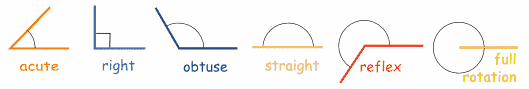Angles

Angles

An angle measures the amount of turn

Names of Angles

As the Angle Increases, the Name Changes:Type of Angle Description
Acute Angle an angle that is less than 90°
Right Angle an angle that is 90° exactly
Obtuse Angle an angle that is greater than 90° but
less than 180°
Straight Angle an angle that is 180° exactly
Reflex Angle an angle that is greater than 180°
Top